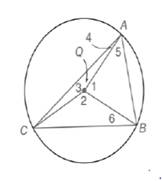Chapter 6.1, Problem 11EElementary Geometry For College St...

7th Edition
Alexander + 2 others
ISBN: 9781337614085

Solutions

Chapter
SectionElementary Geometry For College St...

7th Edition
Alexander + 2 others
ISBN: 9781337614085
Textbook Problem

Given: ⊙ Q  in which m A B ⌢ : m B C ⌢ : m C A ⌢ = 2 : 3 : 4Find: a) m A B ⌢ b) m B C ⌢ c) m C A ⌢ d) m ∠ 1( ∠ A Q B ) e) m ∠ 2 ( ∠ C Q B ) f) m ∠ 3 ( ∠ C Q A ) g) m ∠ 4 ( ∠ C A Q ) h) m ∠ 5 ( ∠ Q A B ) i) m ∠ 6 ( ∠ Q B C ) (HINT): Let m A B ⌢ = 2 x , m B C ⌢ = 3 x , and m C A ⌢ = 4 x . )

To determine

a)

To calculate:

The mAB by using the provided figure.

Explanation

Given:

The provided figure is given below where Q in which mAB:mBC:mCA=2:3:4.

Definition:

Since, the sum of the measures of the consecutive arcs that form a circle is 360o.

Calculation:

Let, the ratio in the form given below:

mAB=2xmBC=3xmCA=4x

Since, the sum of the measures of the consecutive arcs that form a circle is 360o.

To determine

b)

To calculate:

The mBC by using the provided figure.

To determine

c)

To calculate:

The mCA by using the provided figure.

To determine

d)

To calculate:

The m1(AQB) by using the provided figure.

To determine

e)

To calculate:

The m2(CQB) by using the provided figure.

To determine

f)

To calculate:

The m3(CQA) by using the provided figure.

To determine

g)

To calculate:

The m4(CAQ) by using the provided figure.

To determine

h)

To calculate:

The m5(QAB) by using the provided figure.

To determine

i)

To calculate:

The m6(QBC) by using the provided figure.

Still sussing out bartleby?

Check out a sample textbook solution.

See a sample solution

The Solution to Your Study Problems

Bartleby provides explanations to thousands of textbook problems written by our experts, many with advanced degrees!

Get Started

Use the guidelines of this section to sketch the curve. y = arctan(ex)

Single Variable Calculus: Early Transcendentals, Volume I

The difference between population variance and sample variance.

Statistics for The Behavioral Sciences (MindTap Course List)

In Problems 19-26, find the derivative of each function. 25.

Mathematical Applications for the Management, Life, and Social Sciences

The solution to y = y2 with y(1)=13 is: a) y=1x+2 b) y=lnx+13 c) y=12x216 d) y=12x16

Study Guide for Stewart's Single Variable Calculus: Early Transcendentals, 8th